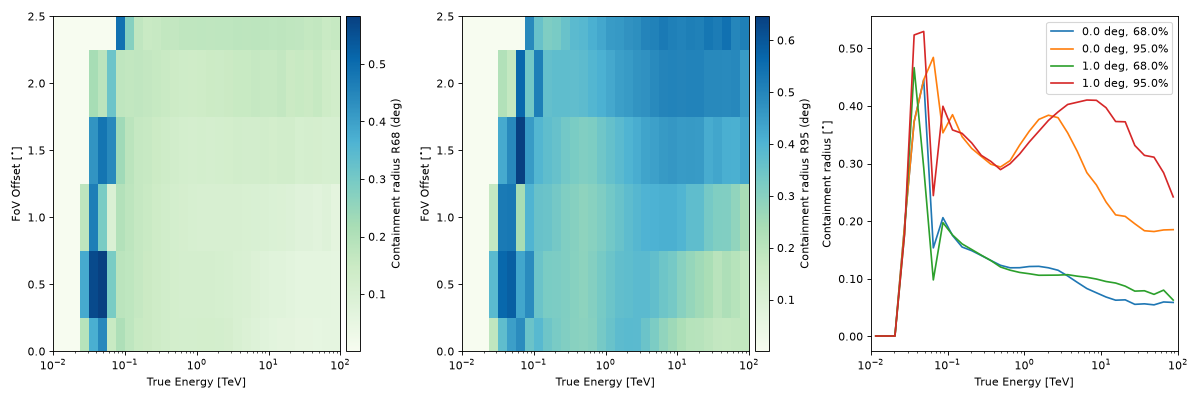As a function of of true energy and offset angle (PSF_TABLE)¶

The PSF3D class represents the radially symmetric probability density of the angular separation between true and reconstructed directions $$\delta p = p_{\rm true} - p$$ (or rad), as a function of true energy and offset angle from the field of view center ($$PSF(E_{\rm true}, \delta p|p_{\rm true})$$ in IRF Theory).

Its format specifications are available in PSF_TABLE.

This is the format in which IACT DL3 PSFs are usually provided, as an example:

"""Plot a PSF from the HESS DL3 data release 1."""
import matplotlib.pyplot as plt
from gammapy.irf import PSF3D

filename = "\$GAMMAPY_DATA/hess-dl3-dr1/data/hess_dl3_dr1_obs_id_020136.fits.gz"
psf.peek()
plt.show()

(png, hires.png, pdf)